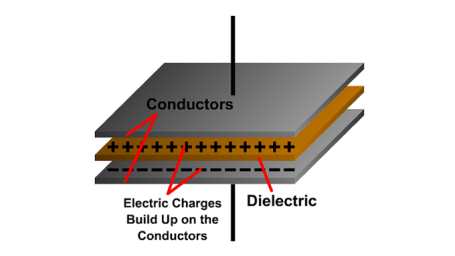Interactive

# Capacitance in AC Circuits

Martech
Updated Jan 21, 2021

After this lesson, you will understand how capacitance reacts in an AC circuit, be able to calculate total values, and understand calculations for capacitive reactance. • Describe the properties of a capacitor and an electrostatic field • Describe how a capacitor charges and discharges • Describe how a capacitor opposes the voltage in a circuit • Identify the values of capacitance • Describe capacitance in series and in parallel • Demonstrate the calculation of capacitive reactance

;